M362K Practice Exam.  Originally given November 12, 1995

This exam covers pretty much the same material as our upcoming exam, with the one difference that variance and covariance will NOT be on our exam.  Solutions to the practice exam are posted below the exam.  Please try to first do the exam WITHOUT looking at the solutions, and only then check your answers.

Problem 1. Pdf's vs. cdf's

A random variable X has the cumulative distribution function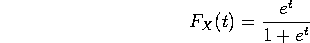a) Is X a discrete random variable, a continuous random variable, or some sort of mixture?

b) Find the probability density function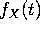.

c) What is the probability that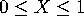? Simplify your answer as much as possible. (Do NOT leave it as some sort of sum or integral).

Problem 2. Expectations and Variances

Let X be a continuous random variable with pdf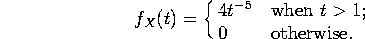a) Compute the mean E(X) and the variance Var(X).

b) Let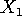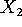and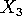be independent random variables, each with the same distribution function as X. Let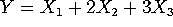. Compute the mean and variance of Y. (Note: You get part credit for expressing E(Y) and Var(Y) in terms of E(X) and Var(X), even if you couldn't do part (a))

Problem 3. Some standard distributions

We flip a fair coin until it comes up heads. Let Y be the number of flips before it first does so (that is, Y=1 if the first toss is heads, Y=2 if the first is tails and the second is heads, and so on) Meanwhile, let X be a Poisson random variable with mean 3.

a) Write down the pdf's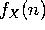and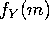.

b) Assuming X and Y are independent, write down the joint pdf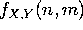.

c) What is the probability that X+Y=13? You may leave your answer as a sum - simplify each term in the sum as much as possible, but DO NOT attempt to do the whole sum.

Problem 4. Joint random variables (2 PAGES!!!!)

Let X and Y be discrete random variables with a joint pdf given by the following table:
(The conversion to HTML left out the key to the table.  The numbers across the top refer to X, while
those along the left refer to Y.  So, for example, the probability that (X=-1 and Y=1) is 7/45.)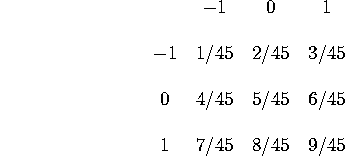[Note: Before starting this problem, you may wish to compute the marginal pdf's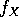and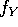]

a) Are X and Y independent random variables? Are the events X=0 and Y=1 independent? What about the events X=-1 and Y=-1?

b) Compute P(X=1|Y=-1), the (conditional) probability of X=1, given that Y=-1.

c) Compute E(X), E(Y) and E(XY). From this, compute the covariance of X and Y.

Problem 1. Pdf's vs. cdf's

A random variable X has the cumulative distribution functiona) Is X a discrete random variable, a continuous random variable, or some sort of mixture?

Since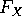is continuous, we are dealing with a CONTINUOUS random variable.

b) Find the probability density function.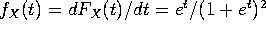.

c) What is the probability that? Simplify your answer as much as possible. (Do NOT leave it as some sort of sum or integral).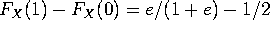(also equals 1/2 - 1/(1+e)).   Since X is continuous, we don't have to worry about the difference between "less than" and "less than or equal".  The probability that X is exactly 0, or exactly 1, is zero.   You could also get the answer by integrating f(t) from 0 to 1.

Problem 2. Expectations and Variances

Let X be a continuous random variable with pdfa) Compute the mean E(X) and the variance Var(X).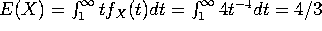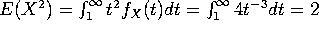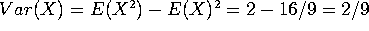.

b) Letandbe independent random variables, each with the same distribution function as X. Let. Compute the mean and variance of Y. (Note: You get part credit for expressing E(Y) and Var(Y) in terms of E(X) and Var(X), even if you couldn't do part (a))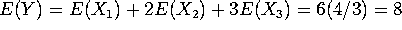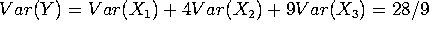.

Problem 3. Some standard distributions

We flip a fair coin until it comes up heads. Let Y be the number of flips before it first does so (that is, Y=1 if the first toss is heads, Y=2 if the first is tails and the second is heads, and so on) Meanwhile, let X be a Poisson random variable with mean 3.

a) Write down the pdf'sand.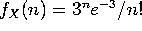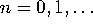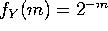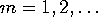.

b) Assuming X and Y are independent, write down the joint pdf.

Since X and Y are independent,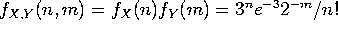for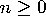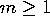.

c) What is the probability that X+Y=13? You may leave your answer as a sum - simplify each term in the sum as much as possible, but DO NOT attempt to do the whole sum.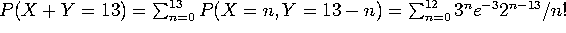. Note that we only get contributions for n up through 12, since n=13 implies m=0, and m is always at least 1.

Problem 4. Joint random variables (2 PAGES!!!!)

Let X and Y be discrete random variables with a joint pdf given by the following table: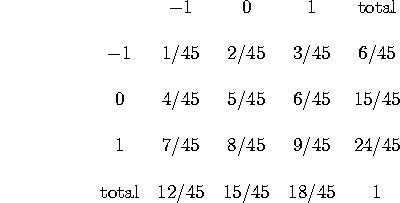a) Are X and Y independent random variables? Are the events X=0 and Y=1 independent? What about the events X=-1 and Y=-1?

No, yes, and no. P(X=0 and Y=1)= 8/45 = P(X=0) P(Y=1), but P(X=-1 and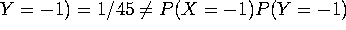. It just takes one counterexample to ruin independence, so X and Y are NOT independent.

b) Compute P(X=1|Y=-1), the (conditional) probability of X=1, given that Y=-1.

P(X=1 and Y=-1)/P(Y=-1) = (3/45)/(6/45) = 1/2

c) Compute E(X), E(Y) and E(XY). From this, compute the covariance of X and Y.

E(X) = -12/45 + 0*(15/45) + 1*(18/45) = 6/45 = 2/15 E(Y) = -6/45 + 0*(15/45) + 1*(24/45) = 18/45 = 2/5 E(XY) = 1/45 - 3/45 - 7/45 + 9/45 = 0, so Cov(X,Y) = E(XY)-E(X)E(Y) = -4/75.